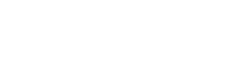سه شنبه ٩ آذر ١٤٠٠مهدی قاسمی

دانشیار

ریاضی کاربردی

دانشکده علوم ریاضی

تلفن: 03832324401

##### مقالات
###### International Journal
1. Farshad Hemati Mehdi Ghasemi Reza Khoshsiar Ghaziani, Numerical solution of the multiterm time‐fractional diffusion equation based on reproducing kernel theory , Numerical Methods for Partial Differential Equations 2020
2. Saeed Vahdati, Mojtaba Fardi, Mehdi Ghasemi, Option pricing using a computational method based on reproducing kernel , Journal of Computational and Applied Mathematics 2018
3. Reza Khoshsiar Ghaziani, Mojtaba Fardi, Mehdi Ghasemi, Solving Large Systems Arising from Fractional Model by Preconditioned Methods , CHIANG MAI JOURNAL OF SCIENCE 2017
4. Mogtaba Fardi Mehdi Ghasemi , Solving nonlocal initial‐boundary value problems for parabolic and hyperbolic integro‐differential equations in reproducing kernel hilbert space , Numerical Methods for Partial Differential Equations 2017
5. Reza Khoshsiar Ghaziani, Mojtaba Fardi, Mehdi Ghasem, Solving multi-order fractional differential equations by reproducing kernel Hilbert space method , Computational Methods for Differential Equations 2016
6. Mogtaba Fardi Reza Khoshsiar Mehdi Ghasemi , The Reproducing Kernel Method for Some Variational Problems Depending on Indefinite Integrals , Mathematical Modelling and Analysis 2016
7. M Ghasemi, E Babolian, ANALYTICAL TREATMENT OF VOLTERRA INTEGRO-DIFFERENTIAL EQUATIONS OF FRACTIONAL DERIVATIVES , Mathematical Researches 2016

##### افتخارات

کلیه حقوق سایت برای دانشگاه شهرکرد محفوظ است